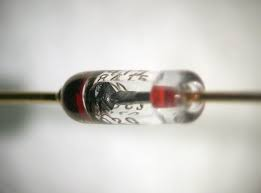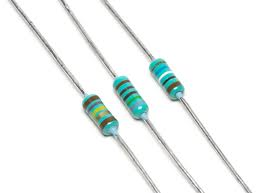# Difference between Diode and Resistor

##### Key Difference: A diode is a type of electrical device that allows the current to move through it in only one direction. It consists of an N-type semiconductor and a P-type semiconductor that are placed together. A resistor is an electric component that is used to provide resistance to current in the circuit. They are mostly used to produce heat or light.A diode can be described as an electronic component that tends to permit the flow of current in one direction. In addition to this, it also inhibits the current flow in the opposite direction. In other words, it is the simplest of two terminal unilateral semiconductor devices. The two terminals of the diodes are known as anode and cathode. It consists of an N-type semiconductor and a P-type semiconductor that are placed together. The Cathode is the P-Type side, and Anode is the N-Type. A diode can be closely related to a switch. One of the most common types of diode is the ‘silicon diode’. It is enclosed in a glass cylinder and also contains a dark band that marks the cathode terminal.

Diodes are often used for rectifying an AC voltage to a DC voltage. There are various types of diodes. For example, a photo diode is the one which allows the current to flow when light strikes it. These types of diodes are greatly used as light detectors. A diode is also known as a Rectifier.Resistors are electronic components that are used to create a precise quantity of resistance in a circuit. Therefore, a resistor can also be termed as a two terminal passive device. They are generally made out of metal wire or carbon. These components are engineered to be able to maintain a stable resistance value. They are available in various shapes and sizes.

If the resistors are connected in a series, the current through each resistor remains the same. However, when the resistors are connected parallelly, then the supplied current is equivalent to the sum of the currents through each resistor. There are numerous types of resistors like Precision Wirewound, NIST Standards, Power Wirewound, Fuse Resistors, Carbon Composition, Carbon Film, Metal Film, Foil, Filament Wound, and Power Film Resistors. Each of these resistors has a useful purpose.

A resistor is generally formed from a type of resistive material and is put into a cylindrical form. Usually, a resistor has a brownish body with several stripes painted on its body. These stripes are actually a code defining the value of the resistor (in ohms).

Comparison between Diode and Resistor:

 Diode Resistor Definition A diode is a type of electrical device that allows the current to move through it in only one direction. A resistor is an electric component that is used to provide resistance to a current in the circuit. These are mostly used intentionally to produce heat or light. Uses Clipping and Clamping - to protect the circuits by limiting the voltage Voltage rectifier – turing AC into DC Voltage Multipliers Non-linear mixing of two voltages Drop voltage Limit current Attenuate signals Act as heaters Act as fuses Furnish electrical loads Divide voltages Types Junction diode (ordinary type) Light emitting (LED) Photodiodes (absorbs light, gives current) Schottky (high speed switch, low turn on voltage, Al. on Silicon) Tunnel (slightly different than junction diodes, negative resistance) Veractor (junction cap. varies with voltage) Zener (special junction diode, use reversed biased) Regular resistors - follow Ohm's Law, which states that the current (I) is proportional to a voltage (V), and where the constant of proportionality is the resistance (R). The equation is V = IR. Variable and non-linear resistors - do not obey Ohm's Law and so are called as non-ohmic. These include potentiometers, varistors, thermistors and photo resistors. Representation in schematic diagram A horizontal line with an arrow pointing to an intersecting vertical line. A resistor is represented by a jagged line.

Images Courtesy: wikipedia.org